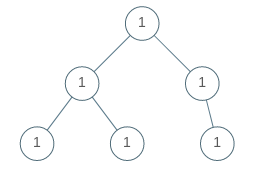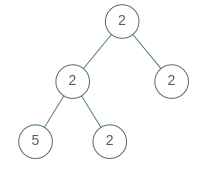# LeetCode: 965. Univalued Binary Tree

## 题目¶

A binary tree is univalued if every node in the tree has the same value.

Return true if and only if the given tree is univalued.

Example 1:```Input: [1,1,1,1,1,null,1]
Output: true
```

Example 2:```Input: [2,2,2,5,2]
Output: false
```

Note:

• The number of nodes in the given tree will be in the range [1, 100].
• Each node's value will be an integer in the range [0, 99].

## 解法¶

```# Definition for a binary tree node.
# class TreeNode:
#     def __init__(self, val=0, left=None, right=None):
#         self.val = val
#         self.left = left
#         self.right = right
class Solution:
def isUnivalTree(self, root: TreeNode) -> bool:
if root is None:
return True
stack = [root]
value = root.val

while stack:
node = stack.pop(0)
if node.val != value:
return False
if node.left is not None:
stack.append(node.left)
if node.right is not None:
stack.append(node.right)

return True
```

## Comments

comments powered by Disqus